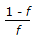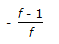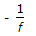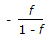# Chemical Engineering - Mass Transfer

21.

Absorption factor method is used to calculate the number of ideal stages, when

 A. operating line lies above the equilibrium line. B. operating line lies below the equilibrium line. C. both operating and equilibrium lines are parallel. D. pressure drop in the column is very high.

Explanation:

No answer description available for this question. Let us discuss.

22.

Flights in a rotary dryer are provided to

 A. lift and shower the solids thus exposing it thoroughly to the drying action of the gas. B. reduce the residence time of solid. C. increase the residence time of the solid. D. none of these.

Explanation:

No answer description available for this question. Let us discuss.

23.

If f = moles of vapour present per mole of feed, then the slope of feed line is (Mcabe-Thiele method)

 A.B.C.D.Explanation:

No answer description available for this question. Let us discuss.

24.

If the solubilities of different components (in a liquid-liquid extraction system) increase with rise in temperature, then the temperature above which they dissolve completely is known as the critical solution temperature (CST or consolute temperature). If solubilities increase with decrease in temperature, then CST is the temperature below which they dissolve completely. If a binary system has no critical solution temperature, it implies that

 A. the system comprises of partially mis-cible liquids. B. the system comprises of miscible liquids. C. the system comprises of an azeotrope. D. on heating, a vapor phase will appear ; while on cooling, a solid phase will appear.

Explanation:

No answer description available for this question. Let us discuss.

25.

The rate of mass transfer is not dependent upon the

 A. degree of dispersion of one fluid in the other. B. interfacial surface exposed between the phases. C. both (a) & (b). D. none of these.